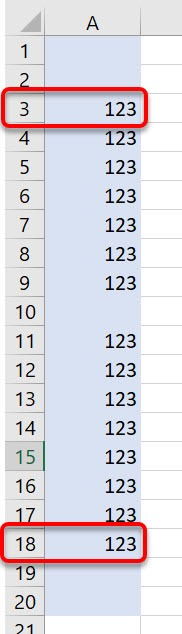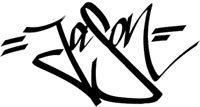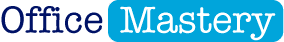# How to calculate the row number of the last populated cell or first populated cell in a range

AUTHOR:  JASON MORRELLminutes remaining

## Context

Imagine you have a range of cells where some of the cells are populated and some are empty, like those shown below.How do you get the row number of the last populated cell or the first populated cell in the range?

That's a question many people have.

In this post, I'll give you four different approaches to achieve this.

## METHOD #1: Using a SUMPRODUCT array formula

This formula will calculate the row number of the last populated cell in the range A1:A20. That's row 18.

###### =SUMPRODUCT(MAX((A1:A20<>"")*ROW(A1:A20)))

Modern versions of Excel are smart enough to recognise when arrays are being used. However, if you are using an older version of  Excel, or a Mac, you need to press CTRL + SHIFT + ENTER which adds braces around the entire formula and tells Excel explicitly that it is an array formula.

Caution: Don't type the braces in yourself. It won't work if you do.

###### {=SUMPRODUCT(MAX((A1:A20<>"")*ROW(A1:A20)))}

Let's break this down.

An array formula is one that allows cell ranges (arrays) to be used within the formula instead of just single cell references.

SUMPRODUCT can use 1 or more arrays as long as they are the same size. It multiplies each corresponding cell of each array, then adds up the values to give a total.

Let's break the formula down.

###### (A1:A20<>"")

This returns TRUE (or 1) for any cell in the range A1:A20 that is populated (I.e. not empty).

###### ROW(A1:A20)

This returns the row number for each cell in the range A1:A20.

###### MAX(Part 1 x Part 2)

On any empty row (e.g. row 1) the test A1:A20<>"" returns FALSE or zero. Any row number multiplied by zero is zero.

On any populated row (e.g. row 3), the test A1:A20<>"" returns TRUE or 1. Any row number multiplied by 1 gives the row number.

The MAX function returns the largest result it finds in the array. In this example, that's 1 x 18 which is row 18.

## METHOD #2: Using a SUM array formula

In the SUMPRODUCT formula above, there is only 1 value being calculated within the SUMPRODUCT brackets - that's a single value 18 which is calculated by the MAX function.

There isn't a second array within the formula so SUMPRODUCT is overkill.

Replacing SUMPRODUCT with SUM works just as well.

## METHOD #3: Using just a MAX array formula

As mentioned above, the MAX function returns one value - 18 - so SUM isn't required either because you are just adding up one value.

This formula works just as well.

## METHOD #4: Using a MAX/IF array formula

I'm going to show you one final method. It's a slightly longer formula but it can be easily adapted to return the row number of the FIRST populated cell instead of the row number of the LAST populated cell.

Here's the formula:.

###### =MAX((IF(A1:A20<>"",ROW(A1:A20),0)))

In the same way, each cell in the array A1:A20 is tested to see if it is populated.

If it is, the row number is returned. If not, zero is returned.

In this example the resulting array is {3, 4, 5, 6, 7, 8, 9, 11, 12, 13, 14, 15, 16, 17, 18 }

The MAX function then selects the  largest number from the returned array - 18.

## Using a MIN/IF array formula to return the row number of the FIRST populated cell

Adapt the formula slighly to:

###### =MIN((IF(A1:A20<>"",ROW(A1:A20),99999999)))

You can use any number instead of 99999999 as long as it is beyond the largest number in the range.

Here is a summary of all the formulas discussed above.

## Sample file

You can download the example file here.

I hope you found this post useful.

Please feel free to post any questions you have into the comments below. And before you leave this page, I want to give you one quick little action item!

ACTION ITEM: Comment below with ONE thing you picked up from this post and share how it has helped, or will help, you. It's good to share your successes because it encourages others and provides an instant boost.

Enjoy the rest of your day!Jason Morrell

Jason loves to simplify the hard stuff, cut the fluff and share what actually works. Things that make a difference. Things that slash hours from your daily work tasks. He runs a software training business in Queensland, Australia, lives on the Gold Coast with his wife and 4 kids and often talks about himself in the third person!

SHAREMaster Microsoft Office
in 30 days or less

more from## How to fix formulas: 21 Fatal Formula Fails (and how to avoid them)

How to fix formulas: 21 Fatal Formula Fails (and how to avoid them)

## How to master the VLOOKUP formula in Excel

How to master the VLOOKUP formula in Excel

## How many rows and columns does Excel have?

How many rows and columns does Excel have?

## Creating New Custom Excel Templates

Creating New Custom Excel Templates

JOIN THE DISCUSSION

{"email":"Email address invalid","url":"Website address invalid","required":"Required field missing"}